# Continuity, modulus of

(diff) ← Older revision | Latest revision (diff) | Newer revision → (diff)

One of the basic characteristics of continuous functions. The modulus of continuity of a continuous functionon a closed interval is defined, with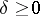, as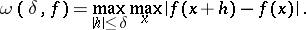The definition of the modulus of continuity was introduced by H. Lebesgue in 1910, although in essence the concept was known earlier. If the modulus of continuity of a functionsatisfies the conditionwhere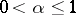, thenis said to satisfy a Lipschitz condition of order.

For a non-negative functiondefined forto be the modulus of continuity of some continuous function it is necessary and sufficient that it has the following properties:,is non-decreasing,is continuous, andOne can also consider moduli of continuity of higher orders,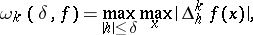where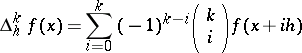is the finite difference of orderof, and moduli of continuity in arbitrary function spaces, for example, the integral modulus of continuity of a functionthat is integrable onto the-th power,: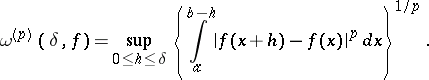(*)

For a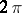-periodic function the integral in (*) is taken over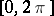.

How to Cite This Entry:
Continuity, modulus of. Encyclopedia of Mathematics. URL: http://encyclopediaofmath.org/index.php?title=Continuity,_modulus_of&oldid=16325
This article was adapted from an original article by A.V. Efimov (originator), which appeared in Encyclopedia of Mathematics - ISBN 1402006098. See original article